# Liu hard sphere equation of state

(diff) ← Older revision | Latest revision (diff) | Newer revision → (diff)

Hongqin Liu proposed a correction to the Carnahan-Starling equation of state which improved accuracy by almost two orders of magnitude :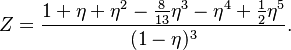$Z = \frac{ 1 + \eta + \eta^2 - \frac{8}{13}\eta^3 - \eta^4 + \frac{1}{2}\eta^5 }{(1-\eta)^3 }.$

The conjugate virial coefficient correlation is given by: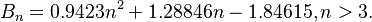$B_n = 0.9423n^2 + 1.28846n - 1.84615, n > 3.$

The excess Helmholtz energy function is given by: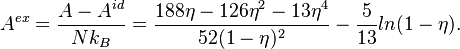$A^{ex} = \frac{ A - A^{id}}{Nk_B}= \frac{ 188\eta - 126\eta^2 - 13\eta^4 }{52(1-\eta)^2} - \frac{5}{13} ln(1-\eta).$

The isothermal compressibility is given by: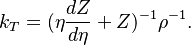$k_T = (\eta\frac{ dZ}{d\eta} + Z)^{-1} \rho^{-1}.$

where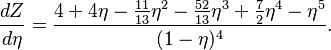$\frac{ dZ}{d\eta} = \frac{ 4 + 4\eta - \frac {11}{13} \eta^2 - \frac{52}{13}\eta^3 + \frac {7}{2}\eta^4 - \eta^5 }{(1-\eta)^4 }.$